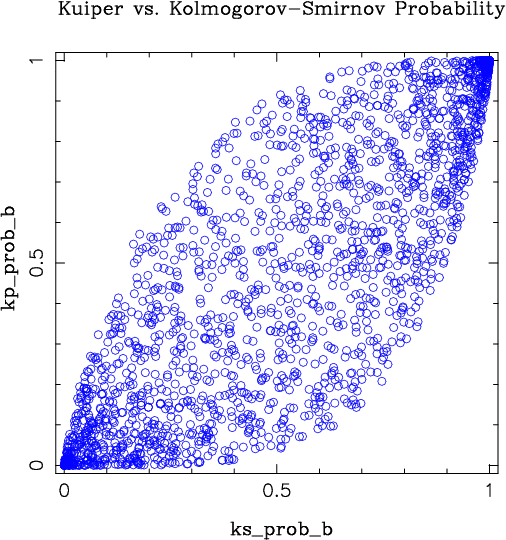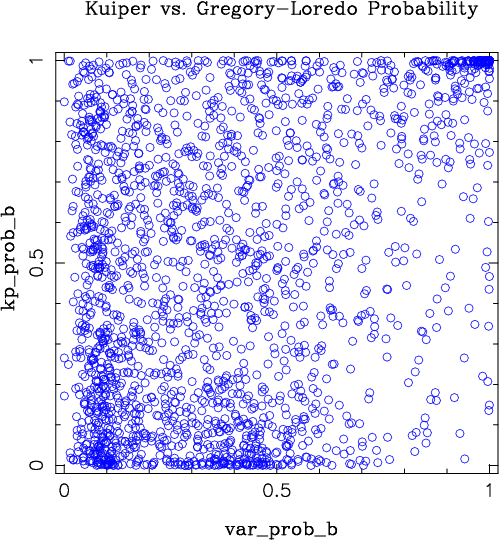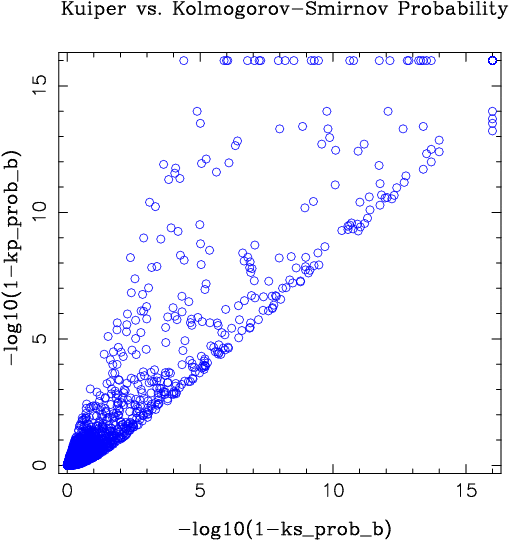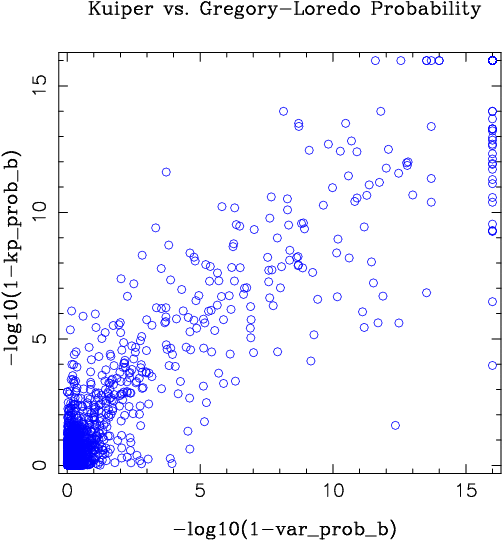## Comparison of the Kolmogorov-Smirnov, Kuiper, and Gregory-Loredo Tests

Different variability tests are known to be sensitive to different kinds of variability. For example, whereas a Fourier based method can be very sensitive to periodic signals, it is less well-suited to detecting a short flare event otherwise interspersed in a light curve dominated by white noise. And even with periodic signals, epoch folding methods might be more sensitive than Fourier methods for folded light curve shapes that are highly non-sinusoidal. As also noted on the Kolmogorov-Smirnov and Kuiper test pages, those two tests have differing sensitivities depending upon the properties of the light curve in question. The Gregory-Loredo test is the third test utilized in the Chandra Source Catalog. It not only has good sensitivity to both aperiodic and periodic signals, but as a byproduct it also provides a "best estimate" of the light curve shape, including estimates of the light curve rate error bars. (An example of this is shown below, to highlight the meaning of these light curves.) Given that different tests can have different sensitivities depending upon the light curve properties, the Chandra Source Catalog provides three separate tests.

In test runs of the catalog pipeline, it was found that when considering the broad band flux, 22% of the sources were considered to be 99% likely variable by at least one of the three methods. Of these likely variable sources, 69% were considered (99% likely) variable by all three methods, and between 75% and 89% were considered (99% likely) variable by a given method. The Gregory-Loredo test was the least likely method to flag a source as variable, whereas the Kuiper test was the most likely to flag a source as variable. Given that the Kuiper and Kolmogorov-Smirnov tests are both comparing the same underlying distributions, albeit with different statistics, the greatest degree of correlation is seen between their respective probabilities, as shown in Figure 1.

Figure 1On the other hand, there is a smaller degree of correlation between either the Kolomogorov-Smirnov or Kuiper test and the Gregory-Loredo test, as shown in Figure 2.

Figure 2Note that the latter figure is dominated by the majority of sources that no tests find significantly variable. A slightly fairer comparison can be made by comparing values for -log10(1-probability). This is shown in Figures 3 and 4 below, which again compare the Kolmogorov-Smirnov and Kuiper tests, and the Kuiper and Gregory-Loredo tests. In general, we see that there is reasonably good correlation among the probabilities for all three tests.

Figure 3Figure 4In the characterization process of the Chandra Source Catalog, further quantitative comparisons among the tests will be made for a variety of light curve types, with varying degrees of variability amplitudes and various mean count rates.